Math - Memory Work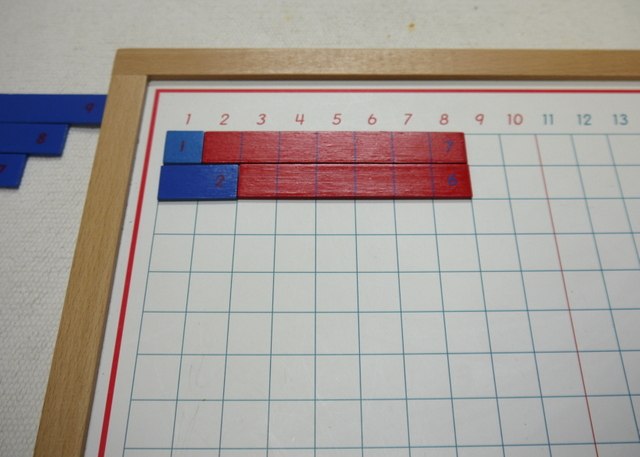LevelPrimary
Age4.5
MaterialsStrip Board
red and blue strips
paper
pencil

## Presentations

### Presentation One

1. Take the material to a mat or table. The number strips can either be placed above or on either side of the board.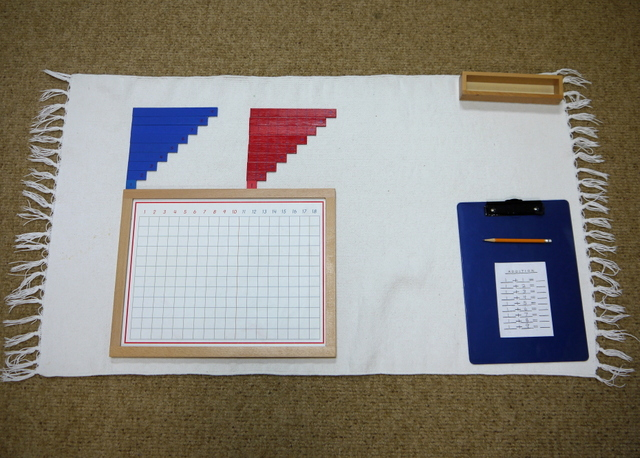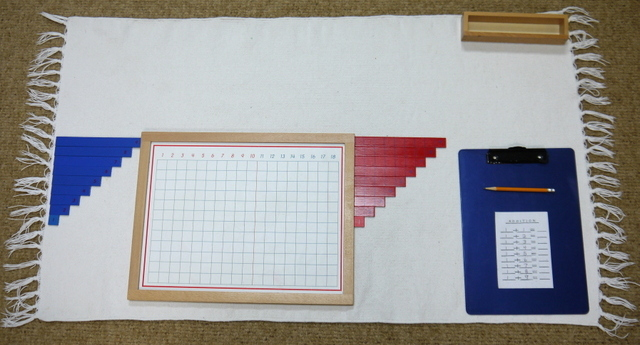2. "1+1"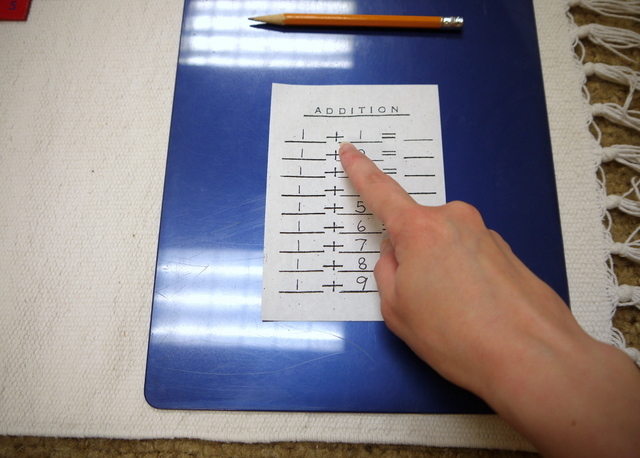3. "One...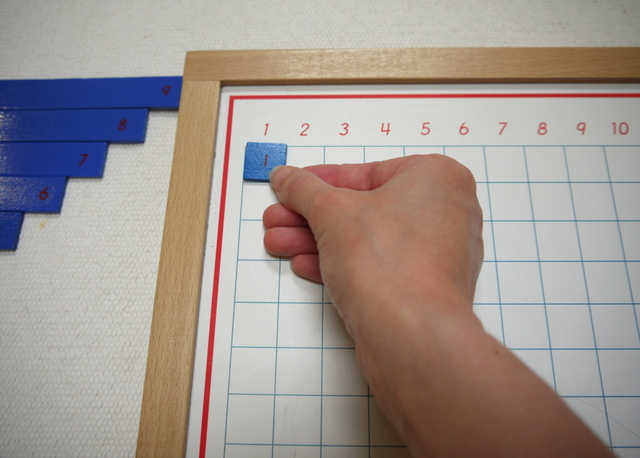4. "...plus one..."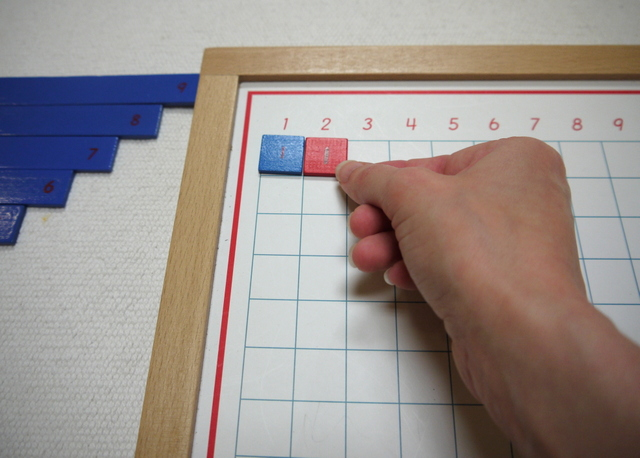5. "...equals two."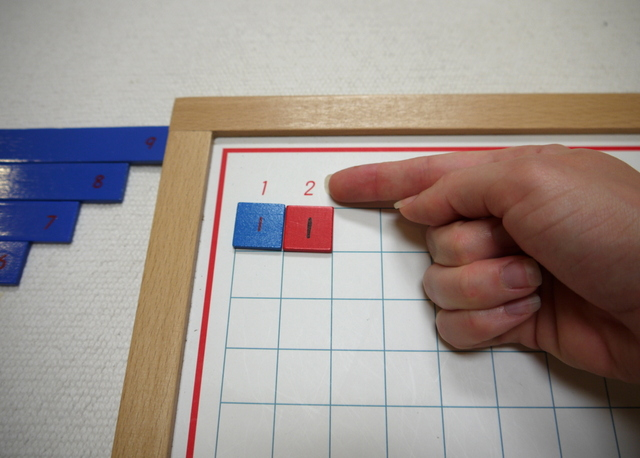6. Write the answer on the paper.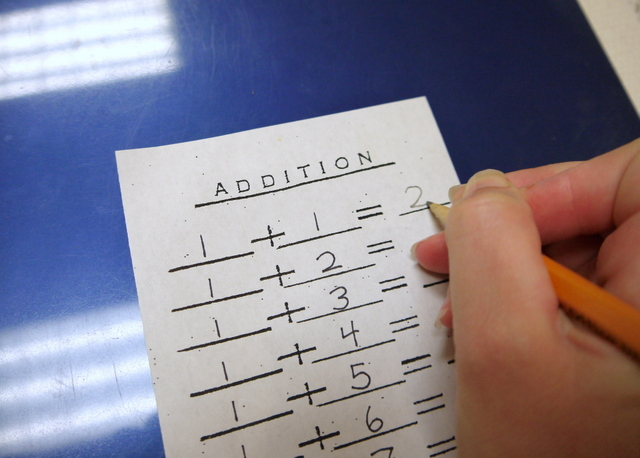7. "One plus two..."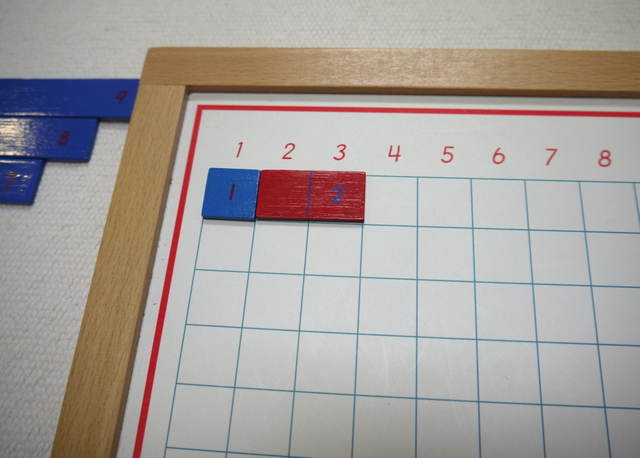8. "...equals three."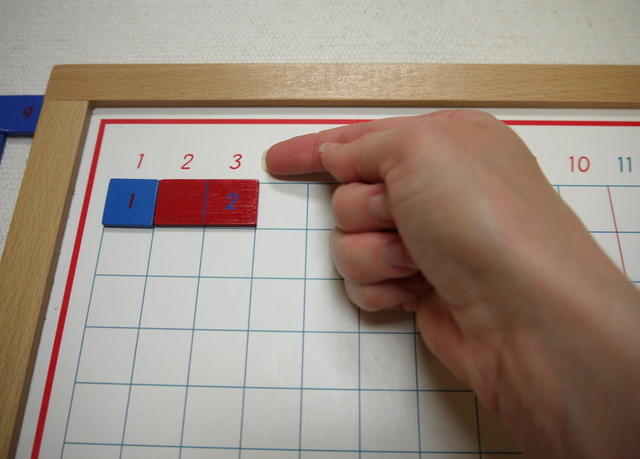9. Write the answer on the paper.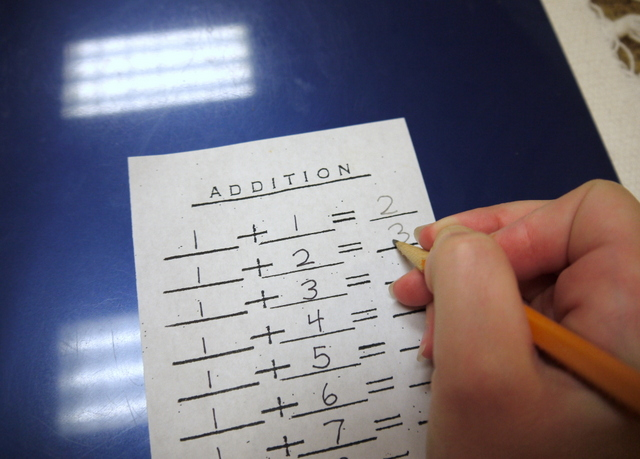10. Do the rest of the problems in the same way.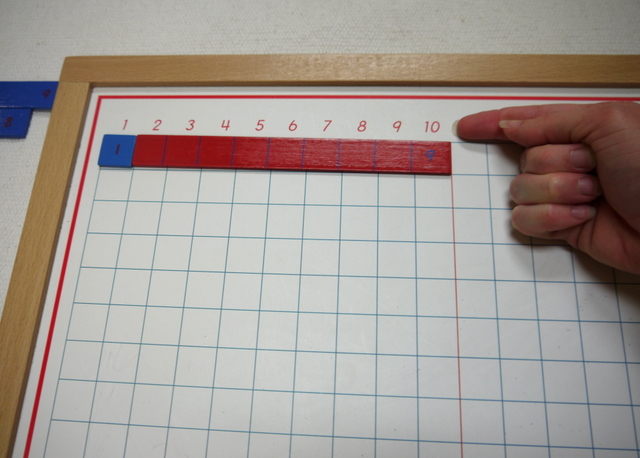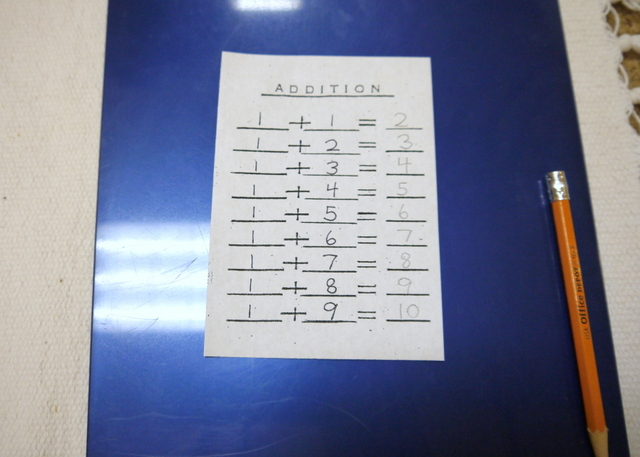1. Bring Addition Control Chart 1.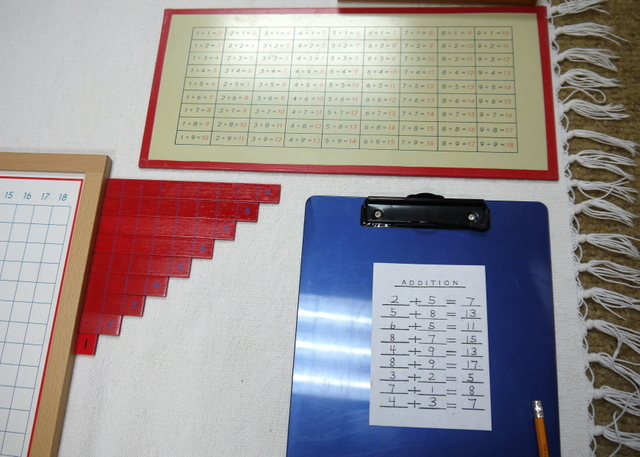2. Find the problem on the paper.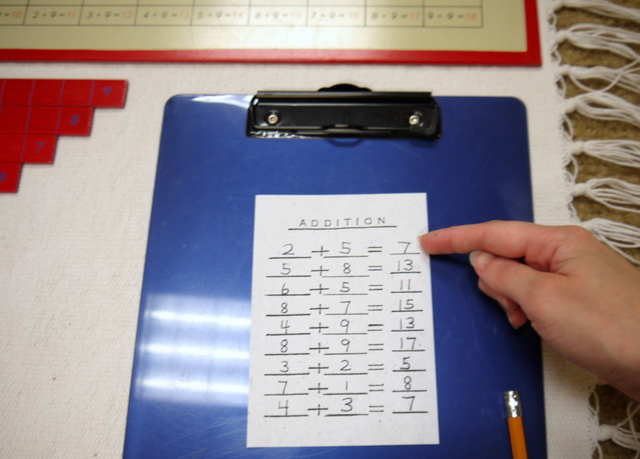3. Find the same problem on the chart. Compare answers.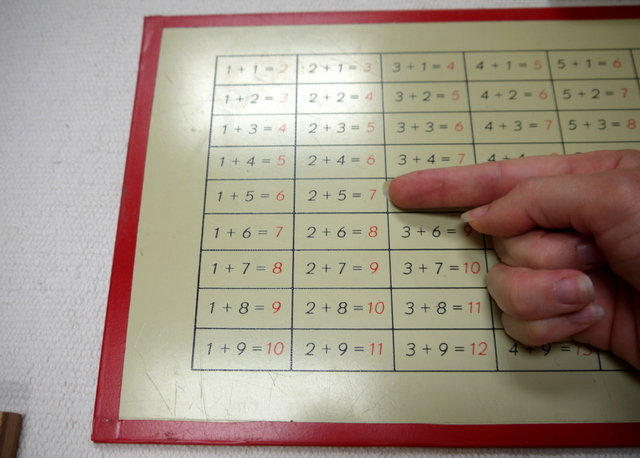### Presentation Three

1. "Let's find all the ways to make 8."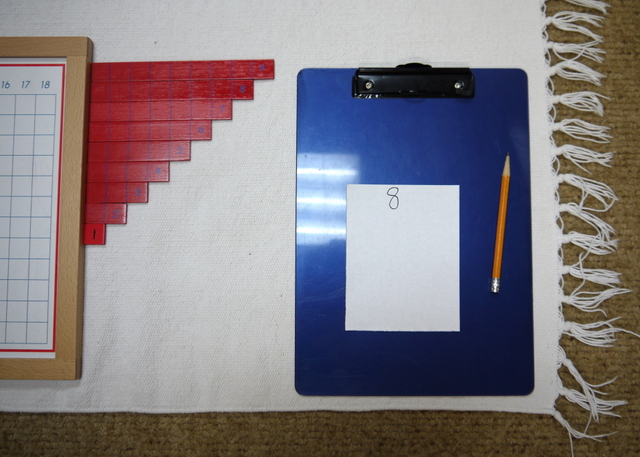2. "1 plus what equals 8?"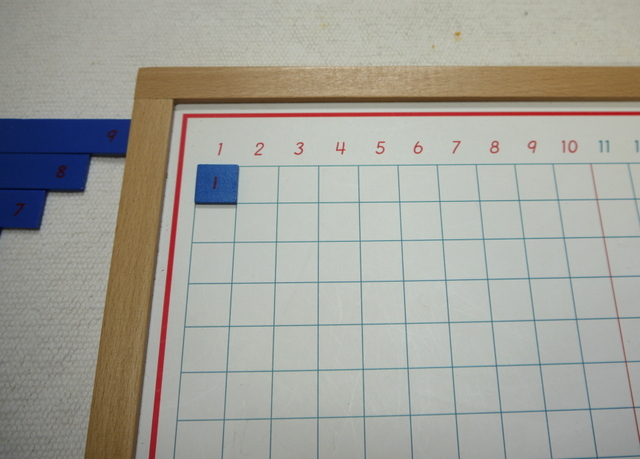3. Count the remaining squares.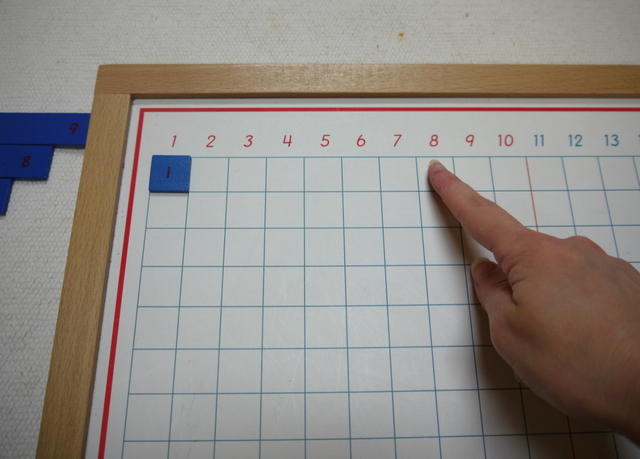4. Put in the appropriate strip.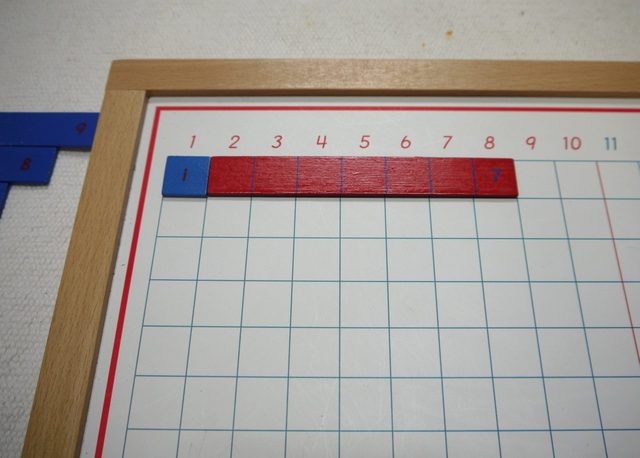5. Write the equation on the paper.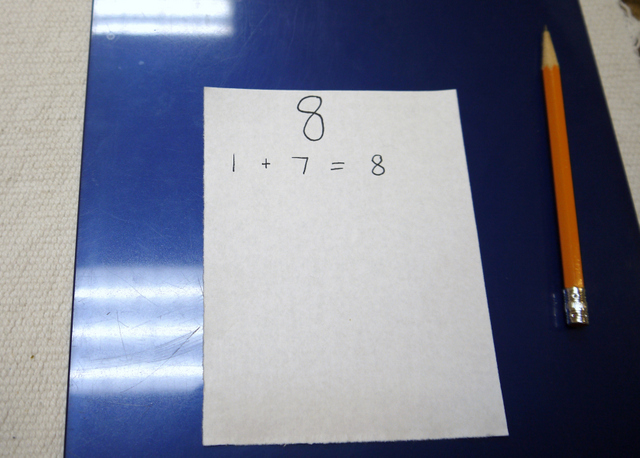6. "2 plus what equals 8?" Count the remaining squares.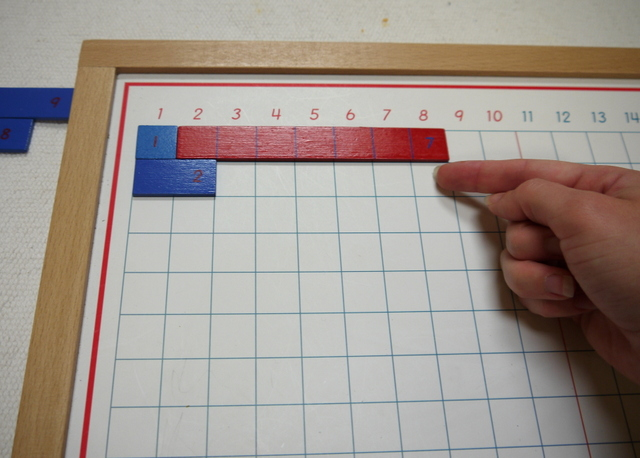7. Put in the appropriate strip.8. Write the equation on the paper.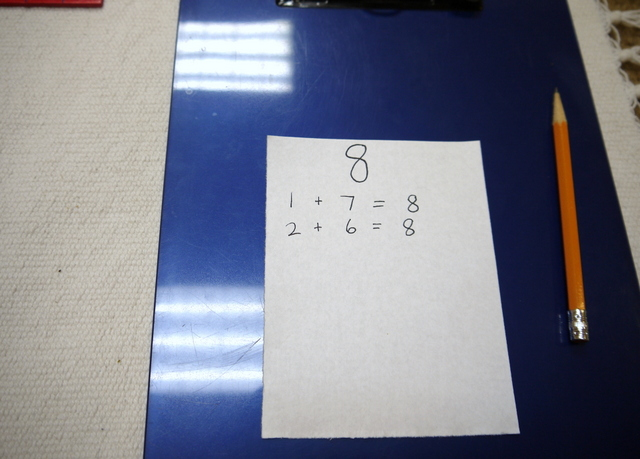9. Do the rest in the same way.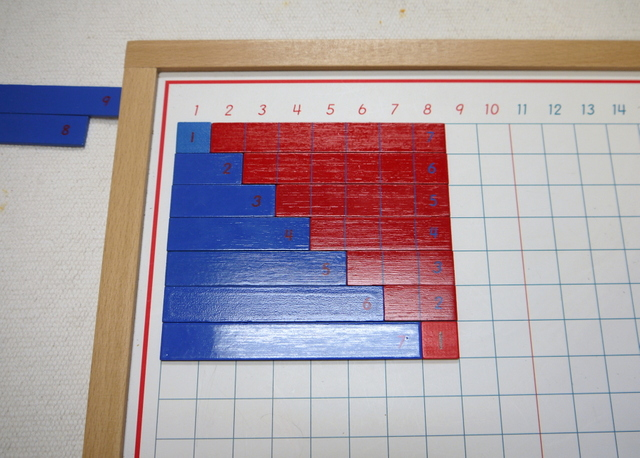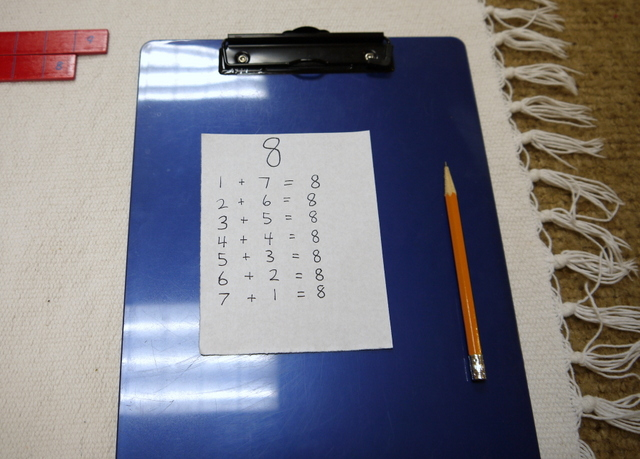## Variations and Extensions

Children can come up with addition problems by rolling two 10-sided dice.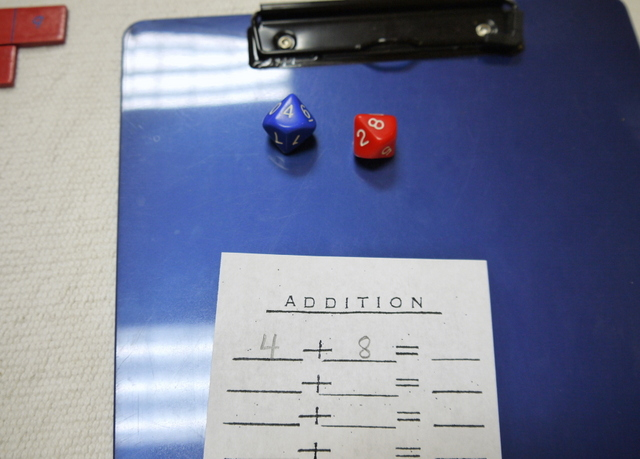## Material

This material is kind of a mess. Why can't the frame come right to the edges of the chart? Almost every kid who tries this the first time slides those strips of wood up against the frame and gets the answers all off by one.